Estimates from PROC GAM

PROC GAM provides the capability to fit both nonparametric and semiparametric models. So that you can better understand the underlying trend of any given factor, PROC GAM separates the linear trend from any general nonparametric trend during the fitting as well as in the final report. This makes it easy to determine whether the significance of a smoothing variable is associated with a simple linear trend or a more complicated pattern.

For example, suppose you want to fit a semiparametric model as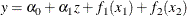The GAM estimate for this model is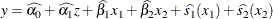where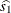and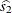are linear-adjusted nonparametric estimates of the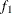and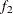effects. The p-values for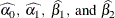are reported in the parameter estimates table.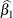and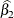are the estimates labeled Linear(x1) and Linear(x2) in the table. The p-values forandare reported in the analysis of deviance table.

Only,, and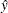are output to the output data set, with the corresponding variable names P_x1, P_x2, and P_y. For Gaussian data, the complete marginal prediction for variable x1 is: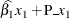If the additive component plots are requested by the ADDITIVE suboption, the additive component for variable x2 is computed as: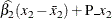where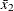is the mean for variable x2.